Worksheet: Triangles

# Worksheet: Triangles - Mathematics (Maths) Class 9

### Multiple Choice Questions

Q1: If AD = BC and ∠ BAD = ∠ ABC, then ∠ ACB is equal to
(a)
∠ABD
(b)
(c)
∠BAC
(d)
∠BDA

Q2: If O is a midpoint of AB and ∠BQO = ∠APO, then ∠OAP is equal to
(a)
∠QPA
(b)
∠OQB
(c)
∠QBO
(d)
∠BOQ

Q3: If △ABC is an isosceles triangle, ∠ B = 650, find ∠ A.
(a)
60º
(b)
70º
(c)
50º
(d)
none of these

Q4: An angle is 140 more than its complement. Find its measure.
(a)
42
(b)
32
(c)
52
(d)
62

Q5: If ABCD is a quadrilateral where AD= CB, AB=CD, and ∠ D= ∠ B, then ∠CAB is equal to
(a)
∠ACD
(b)
(c)
∠ACD
(d)

Q6: If AB ⊥BC and ∠A =∠C, then the correct statement will
(a)
AB ≠ AC
(b)
AB = BC
(c)
(d)
AB = AC

Q7: If AB = AC and ∠ ACD = 1200, find ∠A.
(a)
500
(b)
600
(c)
700
(d)
none of these

Q1: AD and BC are equal perpendiculars to a line segment AB. Show that CD bisects AB.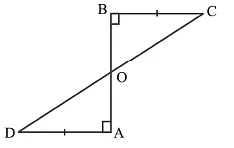Q2: AB is a line segment and P is its mid-point. D and E are points on the same side of AB such that ∠BAD = ∠ABE and ∠EPA = ∠DPB. Show that
(i)
ΔDAP ≌ ΔEBP
(ii)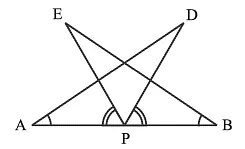Q3: In an isosceles triangle ABC with AB = AC, D and E are points on BC such that BE = CD. Show that AD = AE.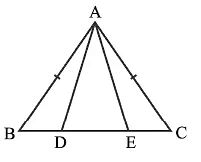Q4: In Figure OA = OB and OD = OC.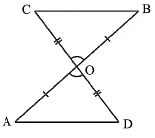Show that
(i) ΔAOD ≅ ΔBOC

Q5: In Fig, AC = AE, AB = AD and ∠BAD = ∠EAC. Show that BC = DE.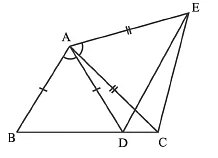Q6: In ΔABC, the bisector AD of ∠A is perpendicular to side BC. Show that AB = AC and ΔABC is isosceles.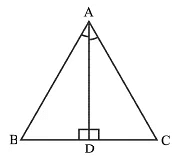Q7: ABC is a triangle in which altitudes BE and CF to sides AC and AB are equal. Show that
(i)
ΔABE ≌ ΔACF
(ii)
AB = AC, i.e., ABC is an isosceles triangle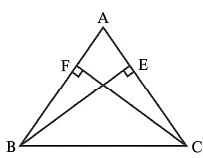The document Worksheet: Triangles | Mathematics (Maths) Class 9 is a part of the Class 9 Course Mathematics (Maths) Class 9.
All you need of Class 9 at this link: Class 9

## Mathematics (Maths) Class 9

42 videos|378 docs|65 tests

## FAQs on Worksheet: Triangles - Mathematics (Maths) Class 9

 1. What are the different types of triangles?Ans. There are three main types of triangles: equilateral triangles, isosceles triangles, and scalene triangles. An equilateral triangle has all three sides and angles equal. An isosceles triangle has two sides and two angles equal. A scalene triangle has all sides and angles unequal.
 2. How can we determine the type of triangle based on its angles?Ans. Triangles can be classified based on their angles. An acute triangle has all three angles less than 90 degrees. A right triangle has one angle equal to 90 degrees. An obtuse triangle has one angle greater than 90 degrees.
 3. What is the Pythagorean Theorem and how is it used to find the length of a side in a right triangle?Ans. The Pythagorean Theorem states that in a right triangle, the square of the length of the hypotenuse (the side opposite the right angle) is equal to the sum of the squares of the lengths of the other two sides. It can be used to find the length of a side in a right triangle by rearranging the formula and solving for the unknown side length.
 4. How can we determine the type of triangle based on its sides?Ans. Triangles can also be classified based on their sides. An equilateral triangle has all three sides equal in length. An isosceles triangle has at least two sides equal in length. A scalene triangle has all sides unequal in length.
 5. How can we calculate the area of a triangle?Ans. The area of a triangle can be calculated using the formula: Area = (base x height) / 2. The base of the triangle is the length of one of its sides, and the height is the perpendicular distance from the base to the opposite vertex. By substituting the appropriate values into the formula, the area of the triangle can be determined.

## Mathematics (Maths) Class 9

42 videos|378 docs|65 testsExplore Courses for Class 9 examSignup to see your scores go up within 7 days! Learn & Practice with 1000+ FREE Notes, Videos & Tests.
10M+ students study on EduRev
Track your progress, build streaks, highlight & save important lessons and more!
Related Searches

,

,

,

,

,

,

,

,

,

,

,

,

,

,

,

,

,

,

,

,

,

;